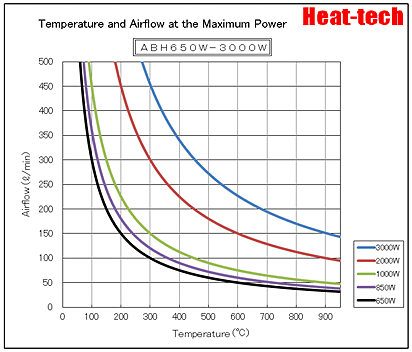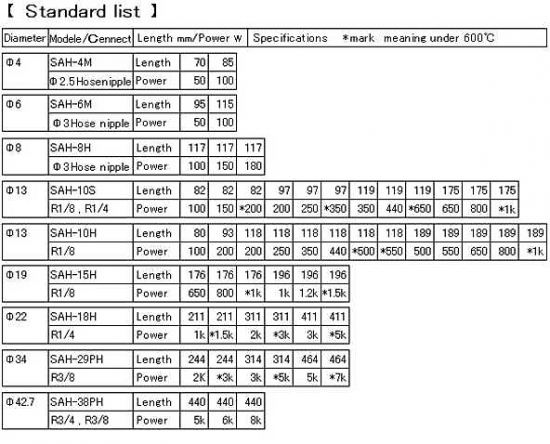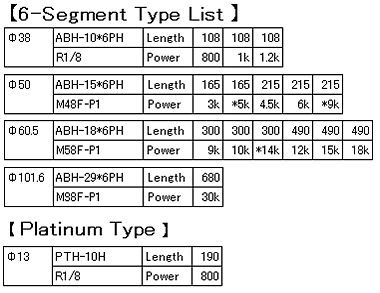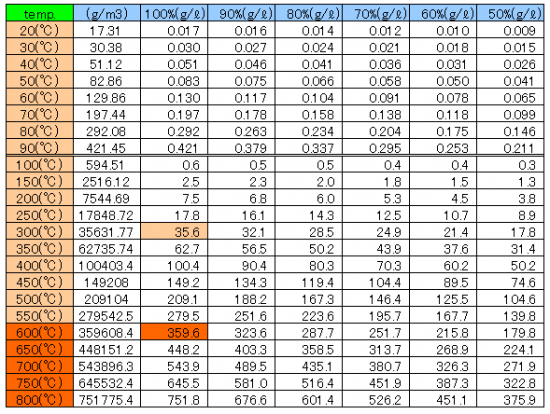# 4-3.Drying speed and temperature of the hot air

### 4-3-1.Features of the hot wind heat source

Dryness with constant temperature and humidity of a hot wind is called the constant drying condition.
If the temperature of a hot air is changed, the relative humidity and specific heat, density, viscosity, and heat conduction will also change.
If the temperature of a hot air is doubled, a coefficient of heat transfer will decrease to 83%.
Therefore, thermal efficiency is 166% even if temperature rises by 200%.

### 4-3-2.Air as a heat source

The air at specific heat at constant pressure of 20 ℃ 1atm is 1.013J / g · K.
The air at specific gravity of 20 ℃ 1atm is 1.2047 kg / m3.
If this is converted into 1ℓ, weight will become 1.2047 g and specific heat will become 1.3 J/K-Cp.

The water vapor is also included, but in the case of the drying process, it remained still as a vapor, it is small enough to be ignored, so it excludes from the equation.

When using heat-Tech Co., Ltd. Air Blow Heater “Model ABH-200v-3kw/29PH/+S“, and was heated to 600 ℃ from room air per minute 200ℓ

200ℓ x(600℃-20℃) x 1.3J/K = 150800J

The air of 200ℓ heated to 600℃ has the quantity of heat 150800 J/min = 2513 J/s.

It need 2454J as latent heat of vaporization and to cause the 1g evaporation of water at 20 ℃ under atmospheric pressure.
When injecting hot air of 600 ℃/3.33ℓ/sec(200ℓ/min) , it will become evaporated in about 60 seconds.

### 4-3-3.The heating capacity of the Air Blow Heater

Power =P

Air flow [L/min] = 1/60F[L/s]

Heat capacity of air = 1.01[J/g/K]

Density of air = 1.2[g/L]

Temperature rise value of airδt = T[K]

*T is Temperature rise value ＝ Output air temperature.－Input air temperature.

Thermal efficiency of the Air Blow Heater = η
Large diameter η = 0.9 (correction factor 10/9 = 1.11)
Medium diameter η = 0.8 (correction factor 10/8 = 1.25)
Small diameter η = 0.7 (correction factor 10/7 = 1.43)

F[L/min.]＝1/60F[L/s]×1.2[g/L]＝0.01666F[L/s]×1.2[g/L] = 0.02F[g/s] P[w＝J/s]＝1.01[J/g/K]×0.02F[g/s]）×Ｔ[K]x η
P =1.01 x 0.02 x 200ℓ x (450-20)℃ x 1.11 = 1930w ≒2000w =2kwPlease refer to the following site table other than the above.

https://heater.heat-tech.biz/air-blow-heater/product-air-blow-heater/5409.html

## Heat-tech Co.,Ltd. Air Blow Heater output table### 4-3-4.Expansion of the gas by heating

PV = nRT by the Charles’s law

Ideal gas equation : pV = nRT
Pressure : p = 1atm = 101.3×10 ^ 5Pa
Volume : V = 1m ^ 3 of air
Number of moles : n = mol
Gas constant : R = 8.31J / mol · K
Temperature : t = 273 + C

### 4-3-5.Carrier gas = Air as the water discharge mechanism

Changes of the saturation water vapor amount by hot air temperatureThere is a maximum value determined by the temperature in the water vapor pressure, can not be present in the state of water vapor at pressures above this value.
This maximum value called the saturation water vapor pressure.

By the Tetens(1930)’s equation E(t)=6.11×10^(7.5t/(t+237.3)
Asks for the saturation water vapor pressure E(t) hPa at the specified temperature t ℃.

The value of the absolute humidity a is the following equation drawn from the equation of state of vapor.